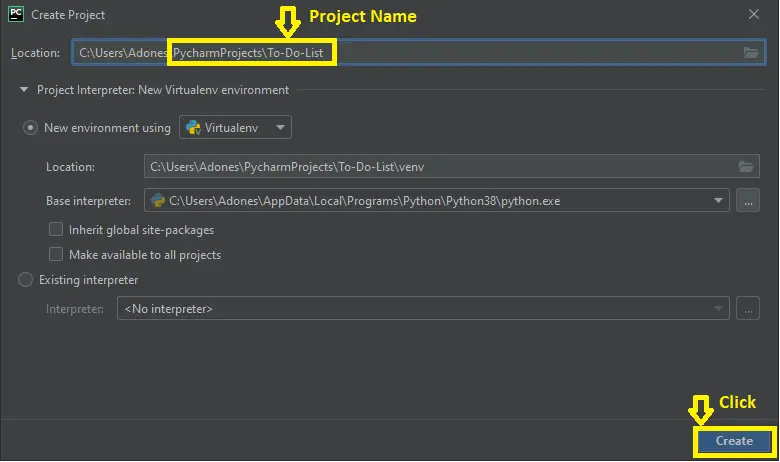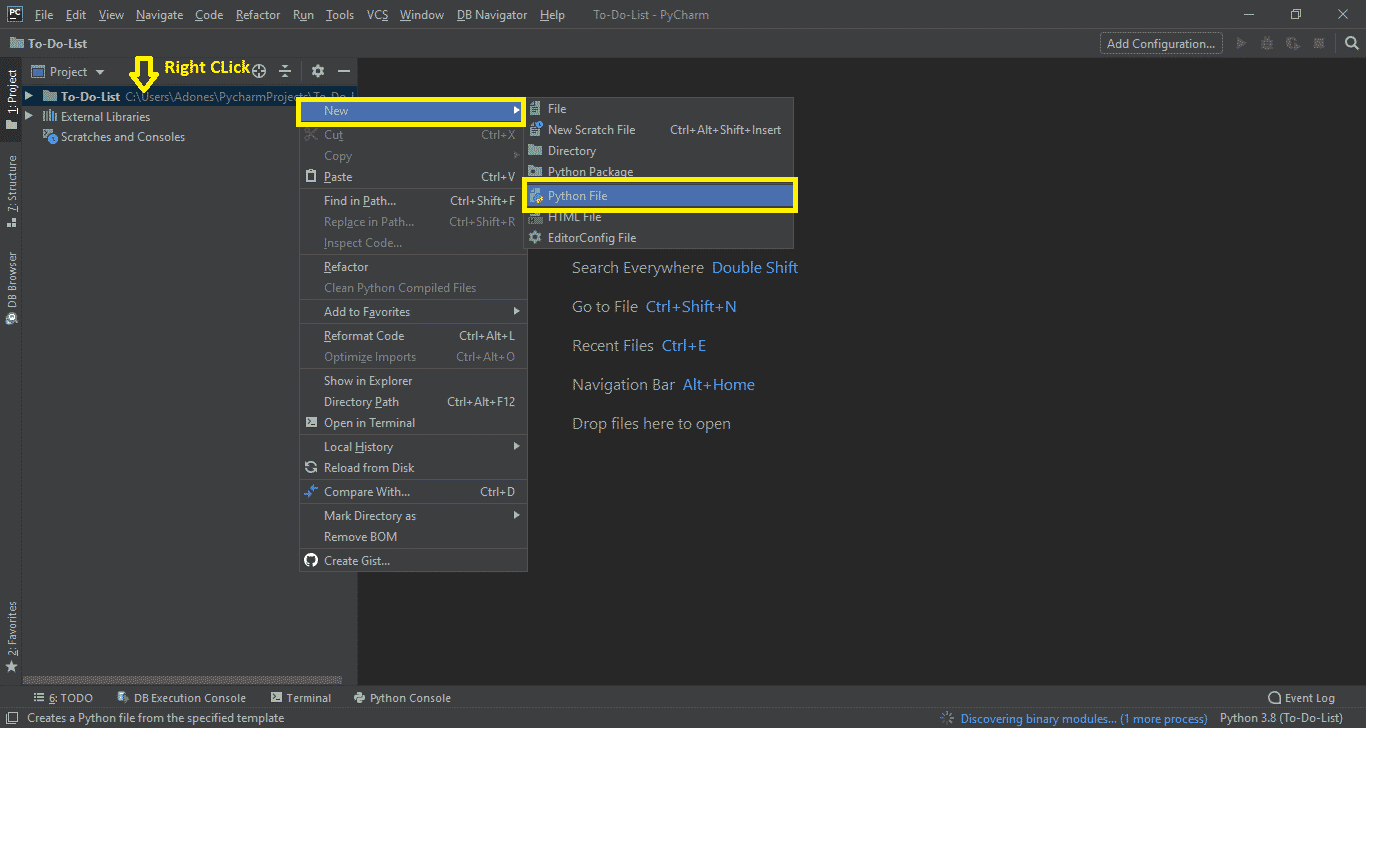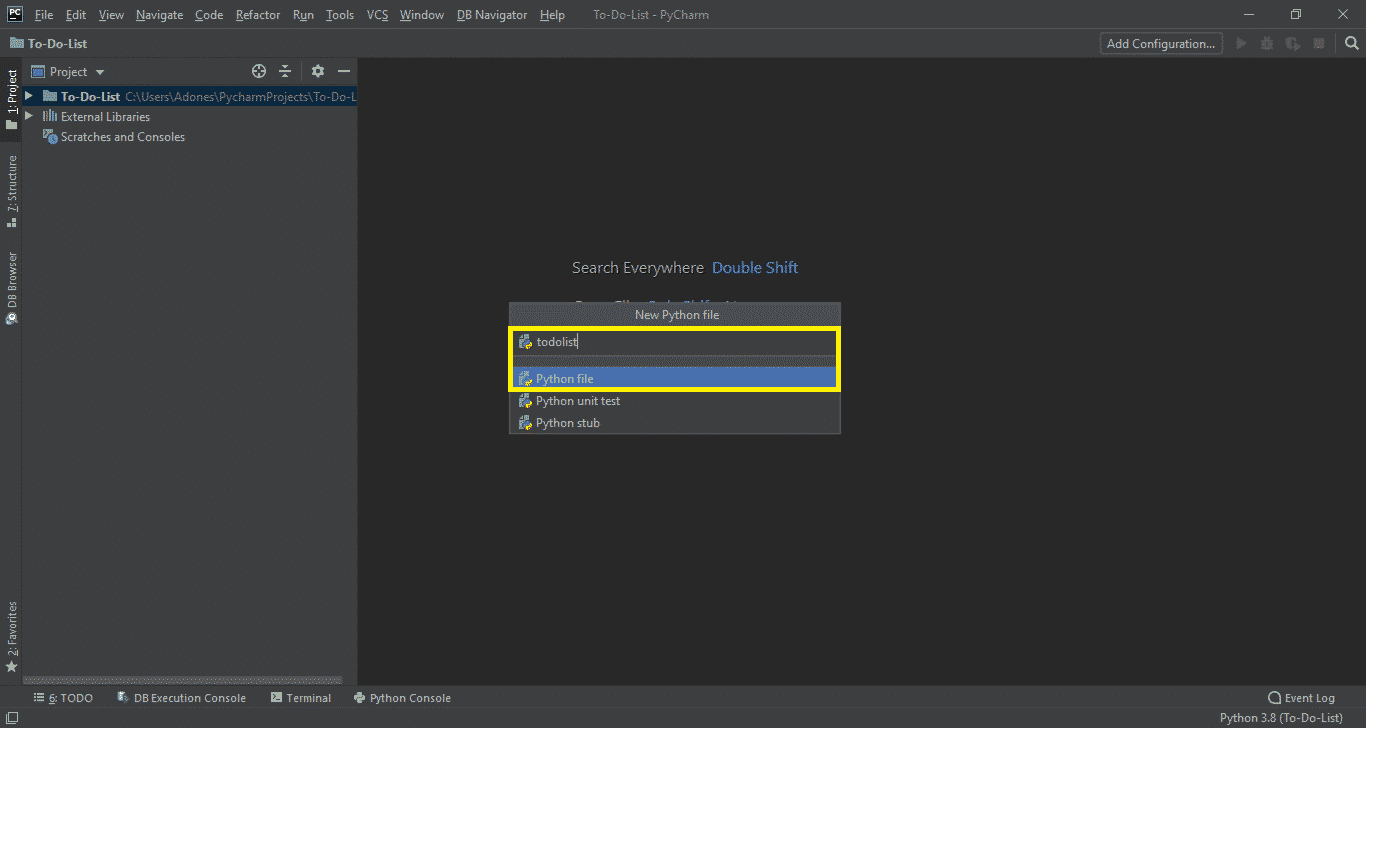# To Do List Project in Python with Source Code

To-Do List Project in Python is a simple desktop project system created using the Python Programming Language, it is designed using tkinter and the project file contains(todolist.py).

This Todo List Application in Python is the simplest way for beginners or college students. If you want more latest projects here. We have a collection of the Best Python Project with Source code free to download for free.

As a beginner, it is easy to Python and would no longer require as many dependencies that you want to download separately.

Python gives the Tkinter toolkit to increase graphical user interface packages.

This article is an open-source that allows the person to download and edit as consistent with their need.

Before you start to create a To-Do List in Python, make sure that you have Pycharm IDE and installed Python on your computer.

By the way, if you are new to Python programming and don’t know what Python IDE to use, I have here a list of the Best Python IDE for Windows, Linux, and Mac OS that will suit you. I also have here How to Download and Install the Latest Version of Python on Windows.

## Steps on How To Create A To-Do List Project in Python.

Time needed: 5 minutes

To-Do List Project in Python with Source Code

• Step 1: Create a Project Name.

First step open the PyCharm IDE and click “File” and select “New Project” and then create a project name after that click the “create” button.• Step 2: Create a Python File.

In the second step after creating a project name, “right” click the project name, and then click “New.” After that choose “Python File“.• Step 3: Name the Python File.

The third step after choosing the Python File name the file “todolist” and then click “Enter.• Step 4: The actual code.

Now you can start coding, you are free to copy the code that being provided below.

## Code Explanation

### 1. Importing Tkinter Module

Tkinter is the standard GUI library for Python. Python when combined with Tkinter provides a fast and easy way to create GUI applications.

``````import tkinter
from tkinter import messagebox``````

In the code above, which is for importing tkinter just write 2 lines of code.

### 2. Module for the Design Main Screen Window

So first of all you have to design the main screen. This display screen has a label to enter tasks to do and enter time(seconds), and for the buttons, it adds task and exit. So let’s see the way to put into effect this.

``````root = tkinter.Tk()
root.geometry("460x480")
root.title("Students to do List Reminder")
root.rowconfigure(0, weight=1)
root.config(bg="blue")

frame = tkinter.Frame(root)
frame.pack()

lbl = tkinter.Label(root, text="Enter Tasks To Do:", fg="white", bg="blue",
font=('Arial', 14), wraplength=200)
lbl_hrs = tkinter.Label(root, text="Enter time (Seconds)", fg="white",
bg="blue", font=('Arial', 14), wraplength=200)
todo = tkinter.Entry(root, width=30, font=('Arial', 14))
time = tkinter.Entry(root, width=15, font=('Arial', 14))
font=('Arial', 16), relief="ridge", bd=5, height=3,
Exit = tkinter.Button(root, text='Exit', fg="white", bg='red', height=3,
font=('Arial Bold', 14), relief="ridge", bd=5, width=30, command=root.destroy)
WorkingList = tkinter.Listbox(root, font=('Arial', 12

))
actual_time()

lbl.place(x=0, y=10, width=200, height=25)
lbl_hrs.place(x=235, y=10, width=200, height=25)
todo.place(x=20, y=40, width=160, height=25)
time.place(x=245, y=40, width=170, height=25)
post.place(x=62, y=80, width=100, height=25)
Exit.place(x=302, y=80, width=50, height=25)
WorkingList.place(x=20, y=120, width=395, height=300)``````

### 3. Module for the Popup Message

A useful thing to be able to do is to have a popup window appear on the screen with a special message.

This can be used to give instant feedback to the user, alert the user to an error, or inform the user that the program completed successfully.

``````def proceed_time(task):

In the code above, which is for showing a popup message after the time seconds is finished off.

### 4. Complete Source Code

``````import tkinter
from tkinter import messagebox
import sys

work = todo.get()
hour = int(time.get())
todo.delete(0, tkinter.END)
time.delete(0, tkinter.END)
todo.focus_set()
if 0 < hour < 999:
updating_record()

clock.start()

def updating_record():
if WorkingList.size() > 0:
WorkingList.delete(0, "end")
WorkingList.insert("end", "" + task + "=======>>> Time left: " + str(task) + " Seconds")

def actual_time():
real_timer.start()
updating_record()

if __name__ == '__main__':
# application
root = tkinter.Tk()
root.geometry("460x480")
root.title("Students to do List Reminder")
root.rowconfigure(0, weight=1)
root.config(bg="blue")

frame = tkinter.Frame(root)
frame.pack()

lbl = tkinter.Label(root, text="Enter Tasks To Do:", fg="white", bg="blue",
font=('Arial', 14), wraplength=200)
lbl_hrs = tkinter.Label(root, text="Enter time (Seconds)", fg="white",
bg="blue", font=('Arial', 14), wraplength=200)
todo = tkinter.Entry(root, width=30, font=('Arial', 14))
time = tkinter.Entry(root, width=15, font=('Arial', 14))
font=('Arial', 16), relief="ridge", bd=5, height=3,
Exit = tkinter.Button(root, text='Exit', fg="white", bg='red', height=3,
font=('Arial Bold', 14), relief="ridge", bd=5, width=30, command=root.destroy)
WorkingList = tkinter.Listbox(root, font=('Arial', 12

))
actual_time()

lbl.place(x=0, y=10, width=200, height=25)
lbl_hrs.place(x=235, y=10, width=200, height=25)
todo.place(x=20, y=40, width=160, height=25)
time.place(x=245, y=40, width=170, height=25)
post.place(x=62, y=80, width=100, height=25)
Exit.place(x=302, y=80, width=50, height=25)
WorkingList.place(x=20, y=120, width=395, height=300)

root.mainloop()
sys.exit("FINISHED")``````

## How To Run The To-Do List Project in Python?

To run this project, you must have installed a Pycharm on your PC (for Windows). This To Do List Project in Python is for educational purposes only!

Step 1: Unzip the file or Extract the file

Step 2: Double-click the todo list

Step 3: The project is ready to run

## Conclusion:

This To Do List is designed using tkinter and the project file contains(todolist.py). This project is good for the student who wants to learn Python programming because this project contains a Graphical User Interface (GUI) and a users friendly.

It is easy to understand and manipulate this project and use it for education purposes only.

## Inquiries

1.2.